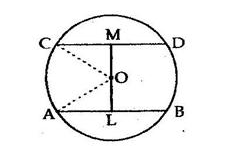# Two parallel chords of lengths 30 cm and 16 cm are drawn on the opposite sides of the centre of a circle of radius 17 cm.

Question:

Two parallel chords of lengths 30 cm and 16 cm are drawn on the opposite sides of the centre of a circle of radius 17 cm. Find the distance between the chords.

Solution:

Let AB and CD be two chords of a circle such that AB is parallel to CD and they are on the opposite sides of the centre.
Given: AB = 30 cm and CD = 16 cm
Draw OL  AB and OM  CD.Join OA and OC.
OA = OC = 17 cm (Radii of a circle)
The perpendicular from the centre of a circle to a chord bisects the chord.

$\therefore A L=\left(\frac{A B}{2}\right)=\left(\frac{30}{2}\right)=15 \mathrm{~cm}$

Now, in right angled ΔOLA, we have:
OA2 = AL2 + LO2
⇒ LO2 = OA2  AL2
LO2 = 172 − 152
LO2 = 289 − 225 = 64
∴ LO = 8 cm

Similarly, $C M=\left(\frac{C D}{2}\right)=\left(\frac{16}{2}\right)=8 \mathrm{~cm}$

In right angled ΔCMO, we have:
OC2 = CM2 + MO2
⇒ MO2 = OC2  CM2
⇒ MO2 = 172 − 82
⇒ MO2  = 289 − 64 = 225
∴ MO = 15 cm

Hence, distance between the chords $=(L O+M O)=(8+15) \mathrm{cm}=23 \mathrm{~cm}$